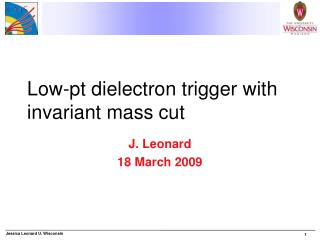DownloadDownload PresentationLow-pt dielectron trigger with invariant mass cut

# Low-pt dielectron trigger with invariant mass cut

Télécharger la présentation## Low-pt dielectron trigger with invariant mass cut

- - - - - - - - - - - - - - - - - - - - - - - - - - - E N D - - - - - - - - - - - - - - - - - - - - - - - - - - -
##### Presentation Transcript

1. Low-pt dielectron trigger with invariant mass cut J. Leonard 18 March 2009

2. Strategy • Trigger path • Characterize current HLT • Use OpenHLT objects • Improve HLT path • Write analyzer to plot quantities used to define HLT electrons • Physics analysis • Characterize reconstructed objects • Write analyzer to keep and plot PAT objects and related information • Plot analysis quantities • Invariant mass, ,  between electrons, etc.

3. Today • Basic distributions for upsilon and j/ signal samples (~80k events each) • PT (or ET), ,  for • All generator-level electrons (gen-level quantities) • L1 isolated and non-isolated candidates (L1 quantities) • HLT “startup-window” and “large-window” electrons (HLT quantities) • Multiplicity of HLT SW and LW electrons • HLT and gen-level electrons in events that pass L1 • L1_DoubleEG1 • L1_DoubleEG5

4. Gen-level, L1, HLT plot: ET (Upsilon

5. Gen-level, L1, HLT plot: ET (J/

6. Gen-level, L1, HLT plot: (Upsilon)

7. Gen-level, L1, HLT plot: (Upsilon)

8. Gen-level electron ET for events that pass L1 (Upsilon)

9. HLT LW electron ET for events that pass L1 (Upsilon)

10. Multiplicity of HLT electrons • J/ • Upsilon

11. Next Steps • Trigger: • Understand plots (esp. wrt L1 bits) • Iterate with Jonathan H about efficiency plots • Don’t look correct • Instrument HLT path to plot quantities (analyzer) • Analysis • Write analyzer, make plots with reconstructed objects